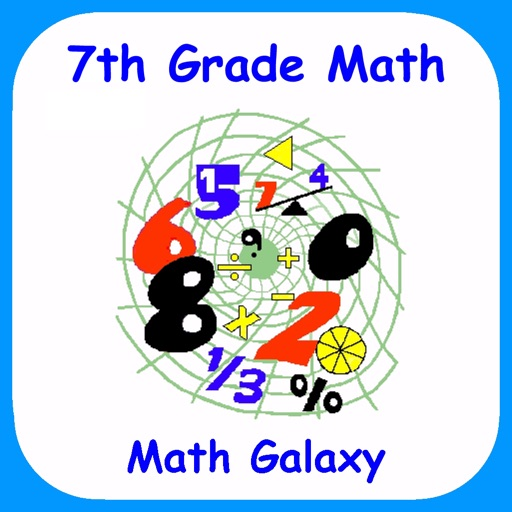## "The French mathematician Jacques S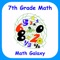# 7th Grade Math - Math Galaxy

by Math Galaxy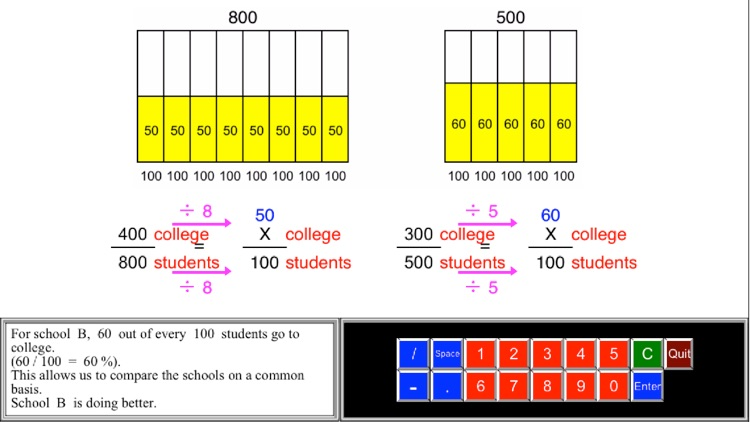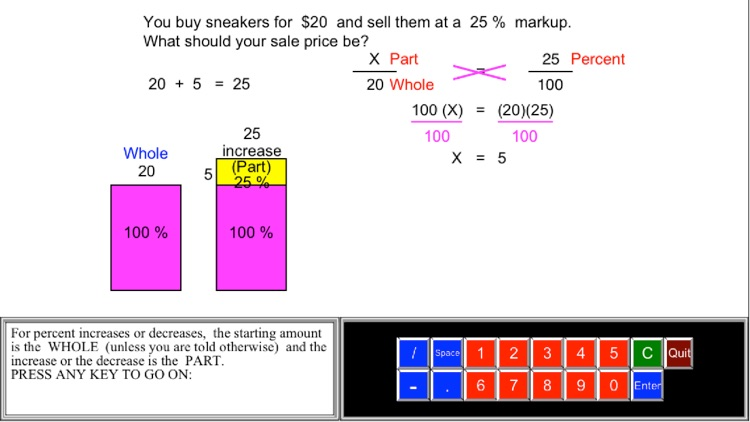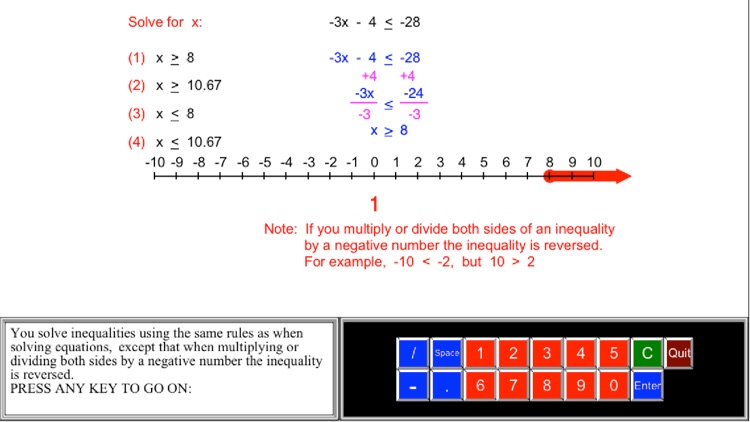"The French mathematician Jacques S. Hadamard found, in a study of 100 leading mathematicians [including Einstein], that the majority of them were primarily visual in their approach."### App Details

Version
3.0
Rating
NA
Size
31Mb
Genre
Education Casual
Last updated
March 26, 2019
Release date
February 24, 2015

### App Screenshots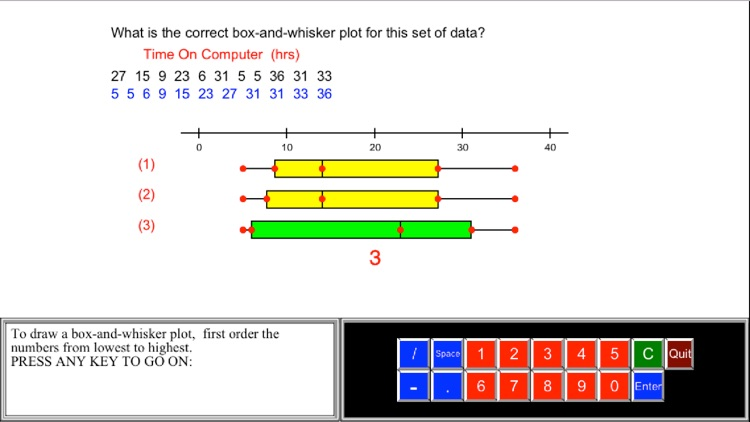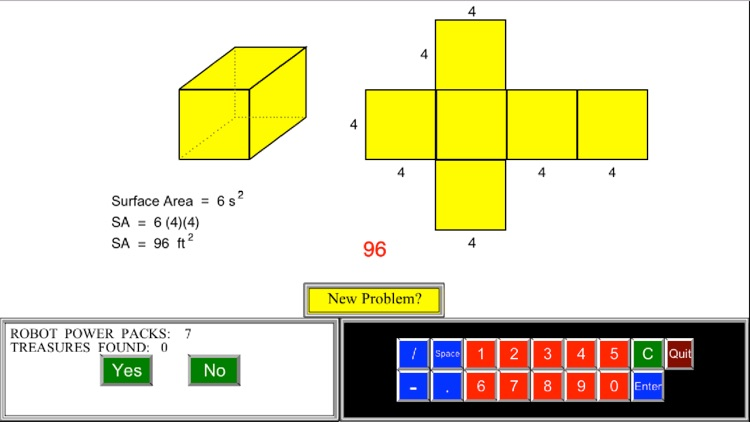### App Store Description

"The French mathematician Jacques S. Hadamard found, in a study of 100 leading mathematicians [including Einstein], that the majority of them were primarily visual in their approach."

Scientific American, Sept., 1984, p. 56.

In seventh grade math the emphasis is on: (1) ratios & proportions, (2) rational and signed numbers, (3) linear equations, (4) scale drawings, area, surface area and volume, and (6) statistical thinking.

Math Galaxy’s Seventh Grade Math covers all of the Common Core math standards for seventh grade as listed below.
The concepts are presented in bite-size pieces with an unlimited supply of practice problems, with numbers chosen randomly.
Illustrations are used throughout to reinforce the concepts.
You are guided through problems step-by-step or can just give final answers.
Scores can be emailed to be printed.
Doing problems earns you robots for the Labyrinth, a maze search game.
Incorporates Schoolwork for classroom management.

RATIOS and PROPORTIONAL RELATIONSHIPS (7.RP)

Analyze proportional relationships and use them to solve real-world and mathematical problems.

Ratios & Proportions
Understanding Ratios (7.RP.1, 2b)
Determining if Proportional (7.RP.2a, 2c)
Ratios on Graphs (7.RP.2b, 2d)
Rates (7.RP.2b, 2d)
Proportions (7.RP.2c)
Review Converting Units (7.RP.2c)
Converting Units (7.RP.2c)

Percents (7.RP.3)
An Explanation of Percents
Percents & Decimals
Fractions, Decimals, Percents
Review Percent Word Problems
Finding %, Part, Whole
Percent Word Problems

RATIONAL NUMBERS (7.NS)

Apply and extend previous understandings of operations with fractions to add, subtract, multiply, and divide rational numbers.

Operations with Signed Numbers (7.NS.1)
Add & Subtract Rational Numbers (7.NS.1c)
Negative # Word Problems (7.NS.3)
Converting Fractions to Decimals (7.NS.2d)
Multiply & Divide Fractions (7.NS.2)
Fraction Word Problems (7.NS.3)

EXPRESSIONS and EQUATIONS (7.EE)

Use properties of operations to generate equivalent expressions.
Solve real-life and mathematical problems using numerical and algebraic expressions and equations.

Simplifying Algebraic Expressions (7.EE.1)
Translating to Algebra (7.EE.2)
Linear Expressions (7.EE.2)
2-Step Equations (7.EE.3, 4a)
Equations with Fractions or Decimals (7.EE.3, 4a)
Equations with Distributive Property (7.EE.3, 4a)
2-Step Inequalities (7.EE.4b)
Linear Equation Word Problems (7.EE.3, 4a)
Inequality Word Problems (7.EE.4b)

GEOMETRY (7.G)

Draw, construct, and describe geometric figures and describe the relationships between them.
Solve real-life and mathematical problems involving angle measure, area, surface area, and volume.

Scale Drawings (7.G.1)
Constructing Figures (7.G.2)
Slicing 3D Figures (7.G.3)
Area Review (7.G.4)
Area & Perimeter (7.G.6)
Complex Areas (7.G.6)
Surface Area with Nets (7.G.6)
Volume Review (7.G.6)
Volume (7.G.6)
Lines, Angles & Polygons (7.G.5)

STATISTICS and PROBABILITY (7.SP)

Use random sampling to draw inferences about a population.
Draw informal comparative inferences about two populations.
Investigate chance processes and develop, use, and evaluate probability models.

Sampling Populations (7.SP.1)
Population Inferences (7.SP.2)
Box-and-Whisker Plots (7.SP.2)
Comparing Populations (7.SP.3, 4)
Probability (7.SP.6, 7)
Compound Events (7.SP.8)

Disclaimer:
AppAdvice does not own this application and only provides images and links contained in the iTunes Search API, to help our users find the best apps to download. If you are the developer of this app and would like your information removed, please send a request to [email protected] and your information will be removed.